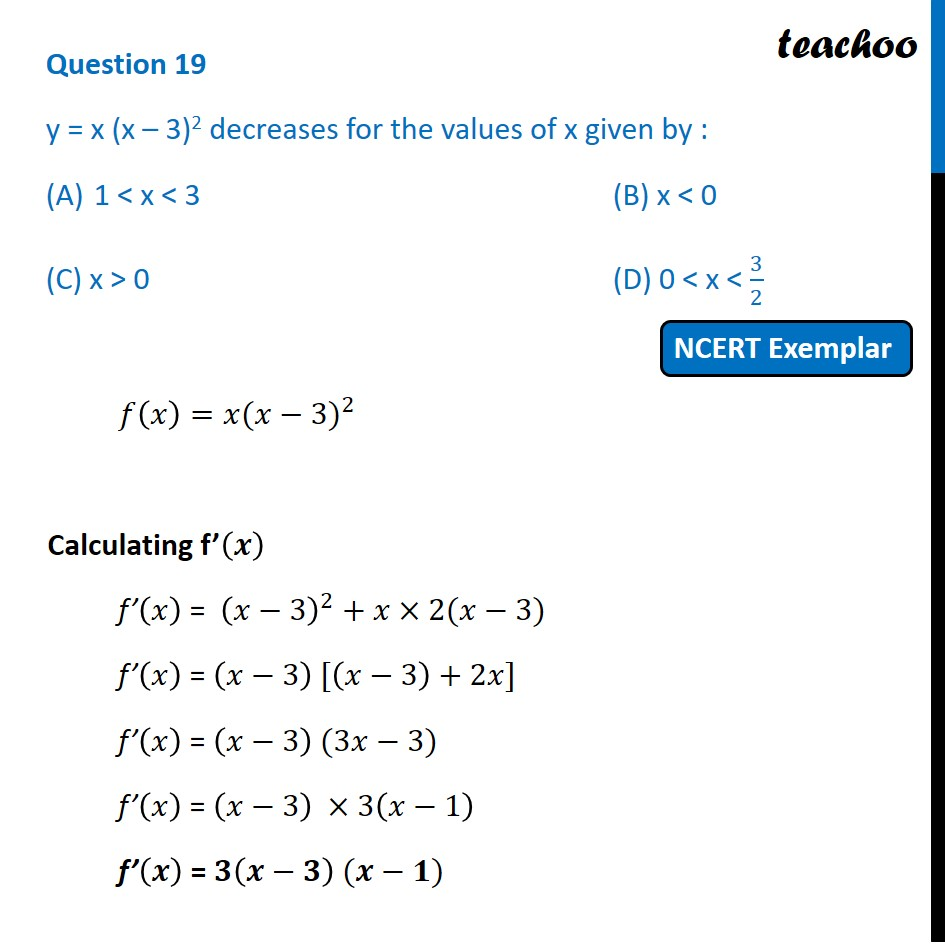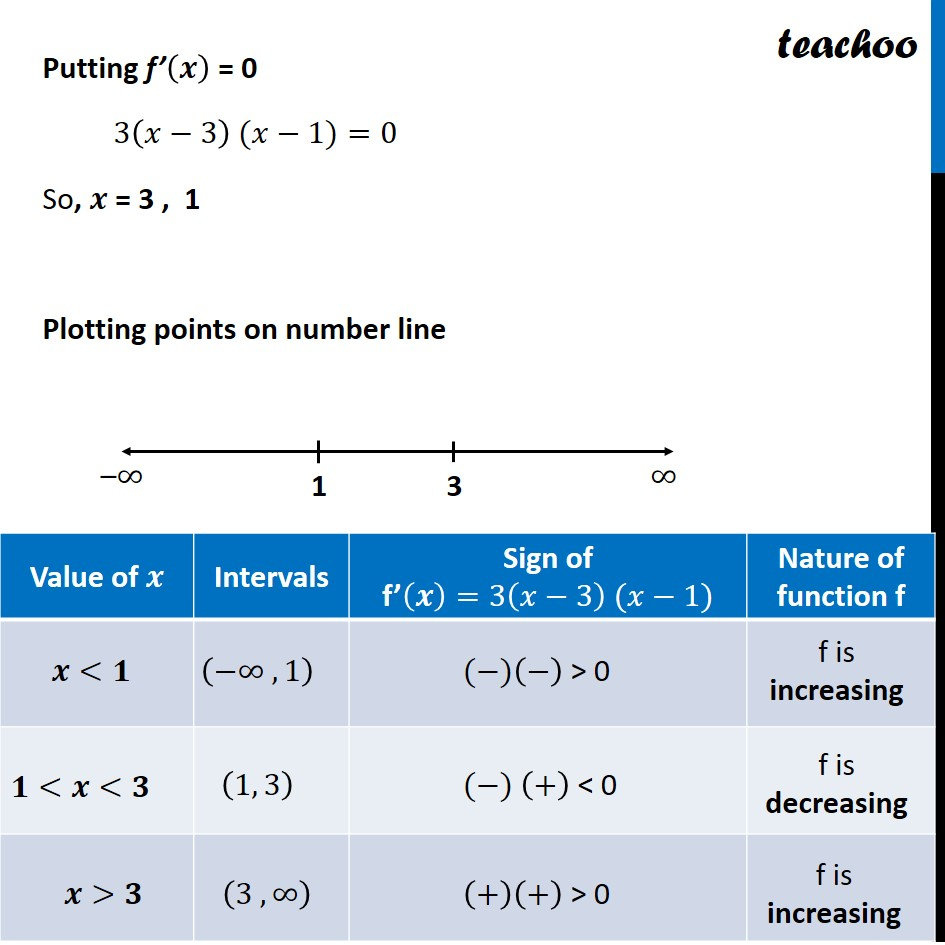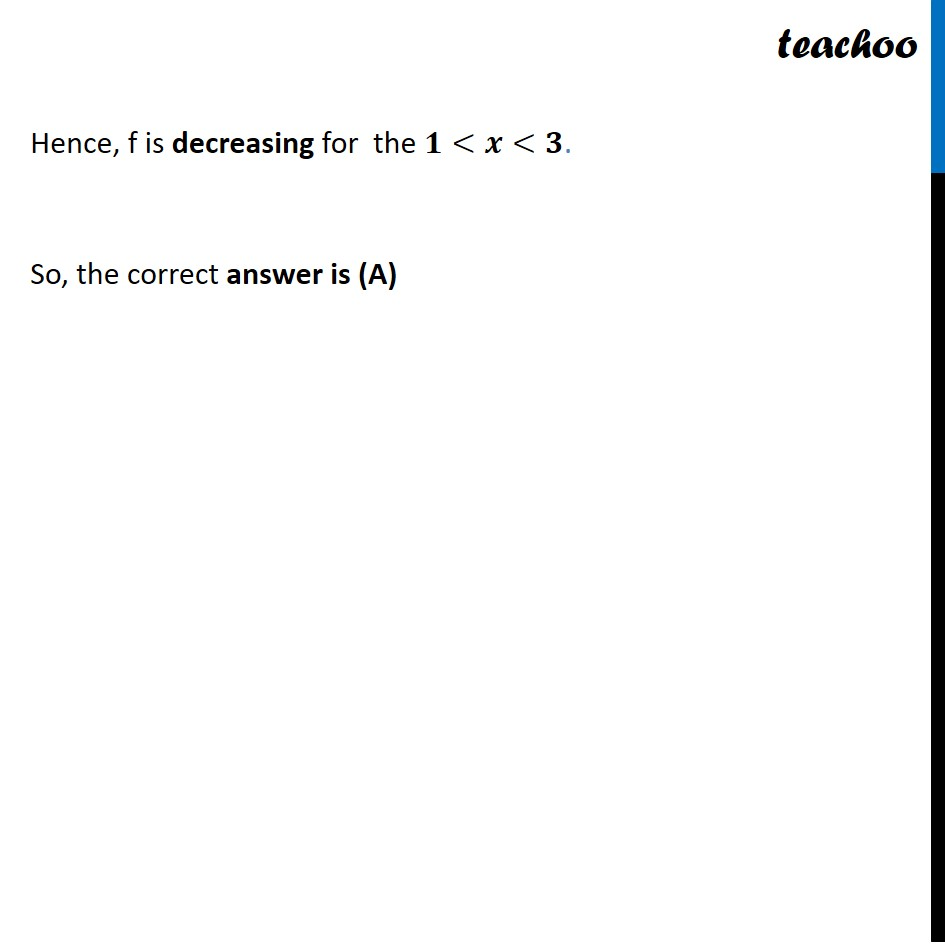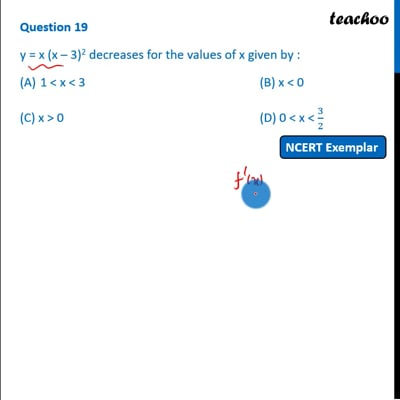NCERT Exemplar - MCQs

Chapter 6 Class 12 Application of Derivatives
Serial order wise

## (D) 0 < x < 3/2

This question is similar to Ex 6.2, 6 - Chapter 6 Class 12 - Application of DerivativesThis video is only available for Teachoo black users

Learn in your speed, with individual attention - Teachoo Maths 1-on-1 Class

### Transcript

Question 6 y = x (x – 3)2 decreases for the values of x given by : 1 < x < 3 (B) x < 0 (C) x > 0 (D) 0 < x < 3/2 𝑓(𝑥)=𝑥〖(𝑥−3)〗^2 Calculating f’(𝒙) f’(𝑥) = (𝑥−3)^2+𝑥×2(𝑥−3) f’(𝑥) = (𝑥−3) [(𝑥−3)+2𝑥] f’(𝑥) = (𝑥−3) (3𝑥−3) f’(𝑥) = (𝑥−3) ×3(𝑥−1) f’(𝒙) = 𝟑(𝒙−𝟑) (𝒙−𝟏) Putting f’(𝒙) = 0 3(𝑥−3) (𝑥−1)=0 So, 𝒙 = 3 , 1 Plotting points on number line Hence, f is decreasing for the 𝟏<𝒙<𝟑. So, the correct answer is (A)